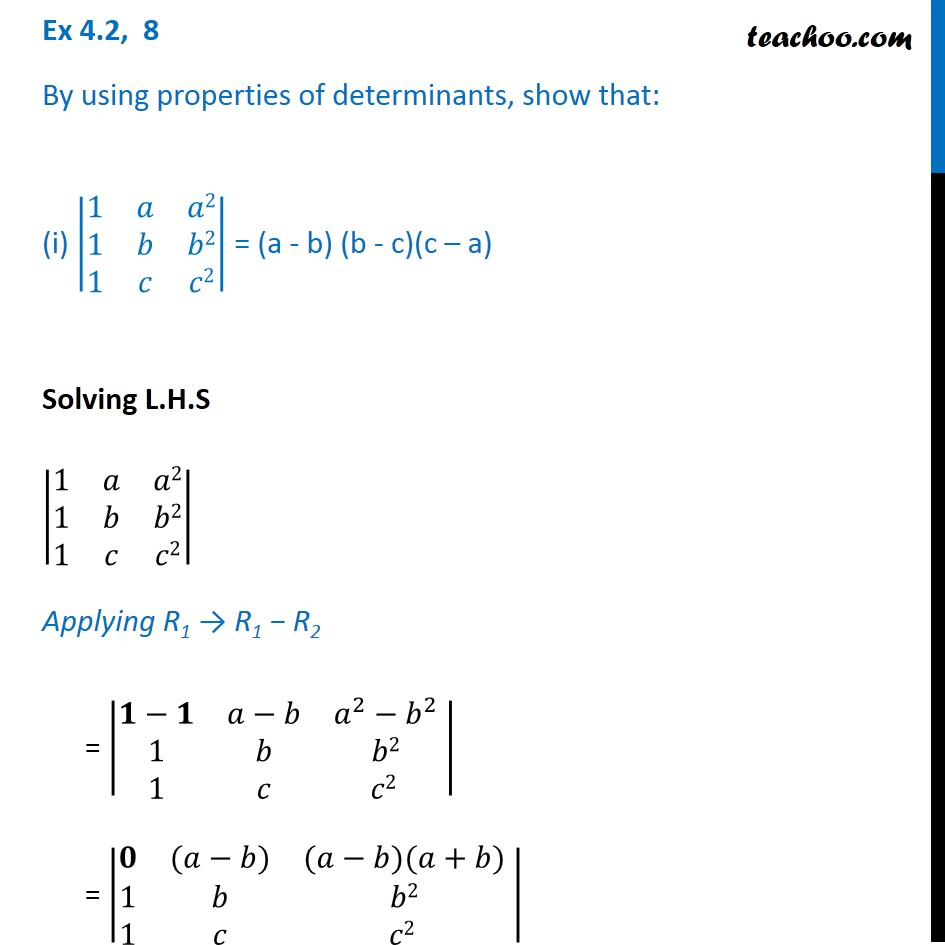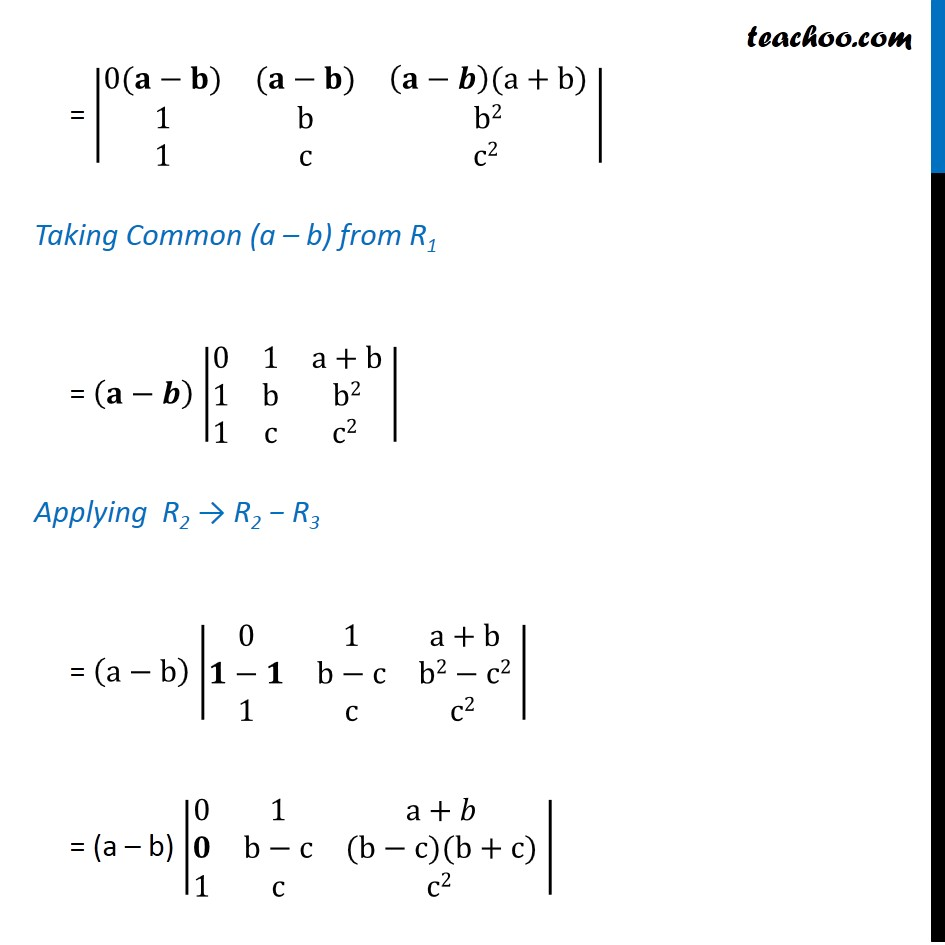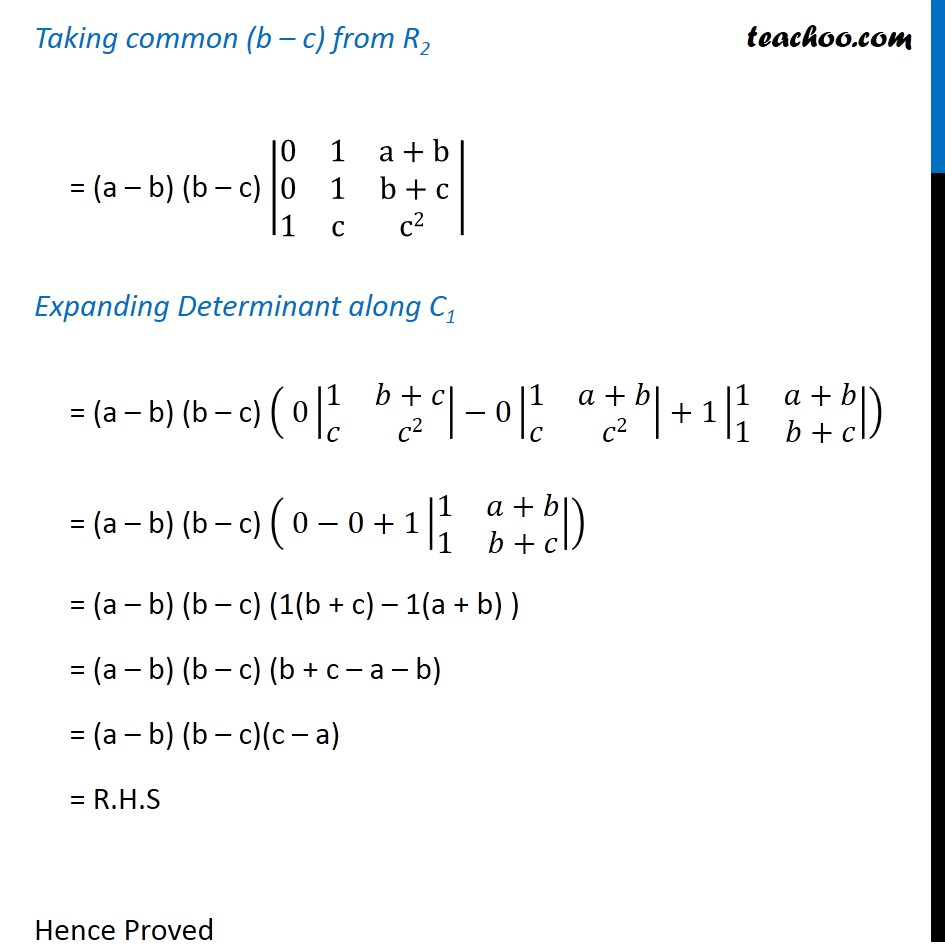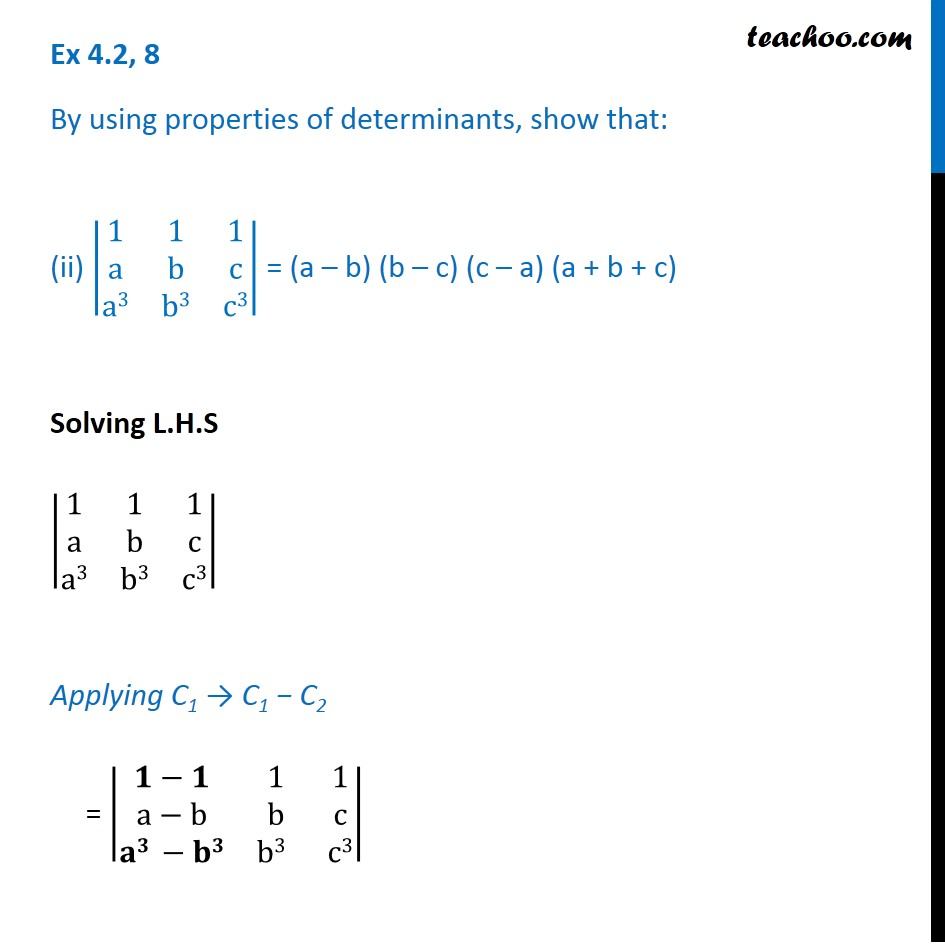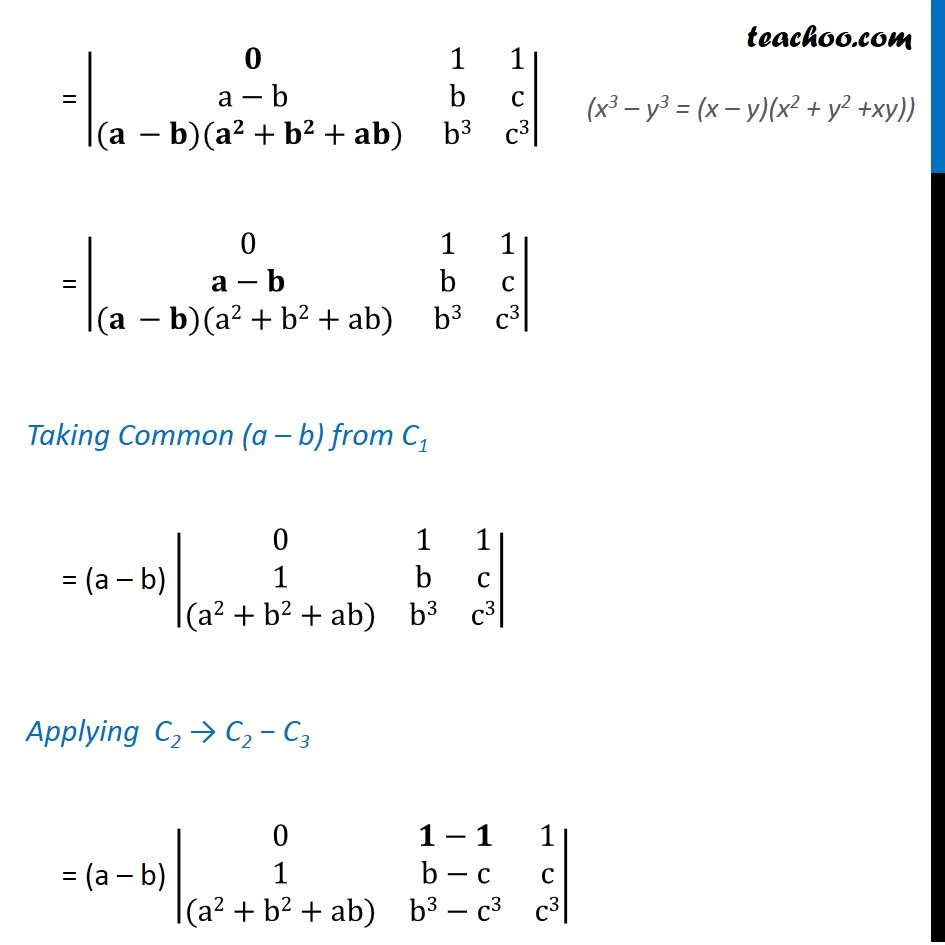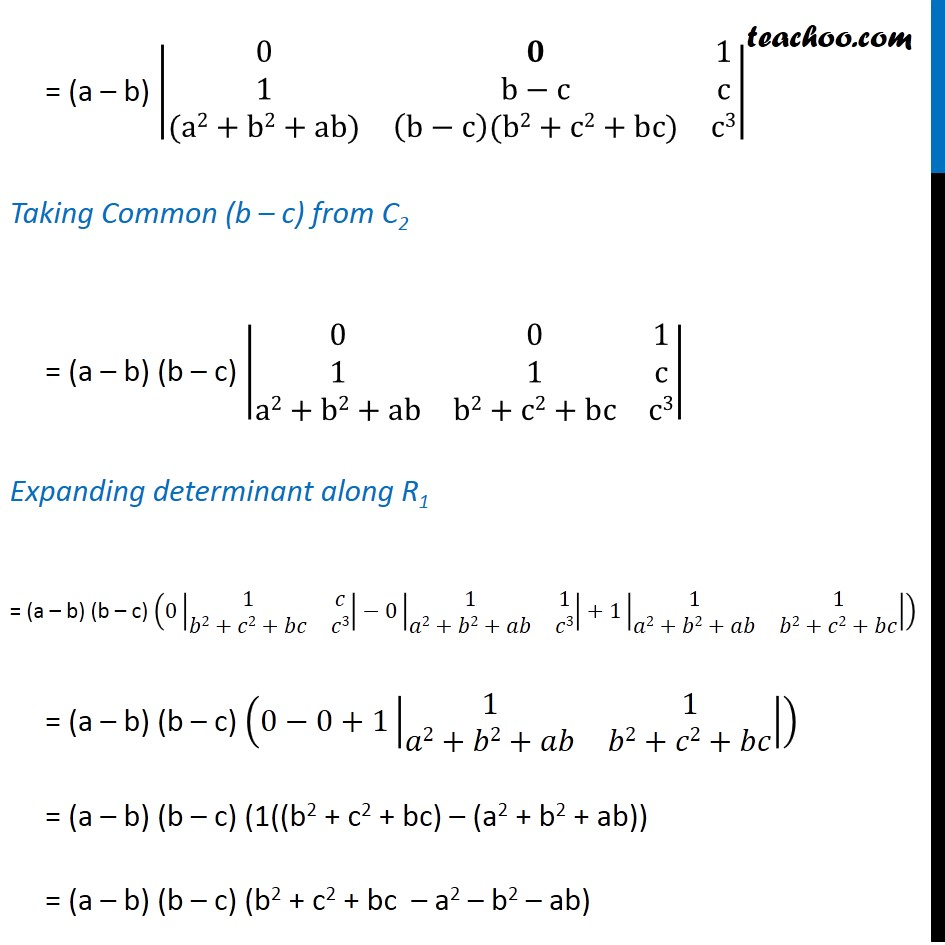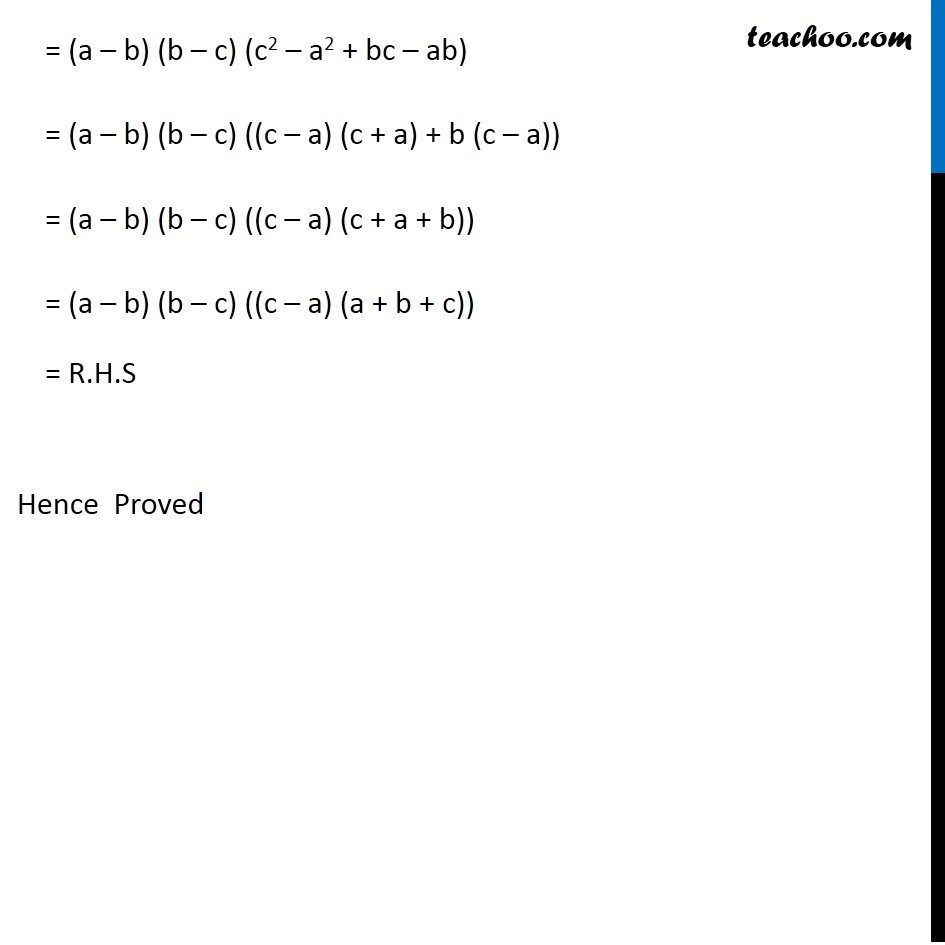Subscribe to our Youtube Channel - https://you.tube/teachoo

1. Chapter 4 Class 12 Determinants
2. Serial order wise
3. Ex 4.2

Transcript

Ex 4.2, 8 By using properties of determinants, show that: (i) |■8(1&𝑎&𝑎2@1&𝑏&𝑏2@1&𝑐&𝑐2)| = (a - b) (b - c)(c – a) Solving L.H.S |■8(1&𝑎&𝑎2@1&𝑏&𝑏2@1&𝑐&𝑐2)| Applying R1 → R1 − R2 = |■8(𝟏−𝟏&𝑎−𝑏&𝑎^2−𝑏^2@1&𝑏&𝑏2@1&𝑐&𝑐2 ) | = |■8(𝟎&(𝑎−𝑏)&(𝑎−𝑏)(𝑎+𝑏)@1&𝑏&𝑏2@1&𝑐&𝑐2 ) | = |■8(0(𝐚−𝐛)&(𝐚−𝐛)&(𝐚−𝒃)(a+b)@1&b&b2@1&c&c2 ) | Taking Common (a – b) from R1 = (𝐚−𝒃) |■8(0&1&a+b@1&b&b2@1&c&c2 ) | Applying R2 → R2 − R3 = (a−b) |■8(0&1&a+b@𝟏−𝟏&b−c&b2−c2@1&c&c2 ) | = (a – b) |■8(0&1&a+𝑏@𝟎&b−c&(b−c)(b+c)@1&c&c2 ) | Taking common (b – c) from R2 = (a – b) (b – c) |■8(0&1&a+b@0&1&b+c@1&c&c2 ) | Expanding Determinant along C1 = (a – b) (b – c) ( 0|■8(1&𝑏+𝑐@𝑐&𝑐2)|−0|■8(1&𝑎+𝑏@𝑐&𝑐2)|+1|■8(1&𝑎+𝑏@1&𝑏+𝑐)|) = (a – b) (b – c) ( 0−0+1|■8(1&𝑎+𝑏@1&𝑏+𝑐)|) = (a – b) (b – c) (1(b + c) – 1(a + b) ) = (a – b) (b – c) (b + c – a – b) = (a – b) (b – c)(c – a) = R.H.S Hence Proved Ex 4.2, 8 By using properties of determinants, show that: (ii) |■8(1&1&1@a&b&c@a3&b3&c3)| = (a – b) (b – c) (c – a) (a + b + c) Solving L.H.S |■8(1&1&1@a&b&c@a3&b3&c3)| Applying C1 → C1 − C2 = |■8(𝟏−𝟏&1&1@a−b&b&c@𝐚𝟑 −𝐛𝟑&b3 &c3)| = |■8(𝟎&1&1@a−b&b&c@(𝐚 −𝐛)(𝐚𝟐+𝐛𝟐+𝐚𝐛) &b3&c3)| = |■8(0&1&1@𝐚−𝐛&b&c@(𝐚 −𝐛)(a2+b2+ab) &b3&c3)| Taking Common (a – b) from C1 = (a – b) |■8(0&1&1@1&b&c@(a2+b2+ab)&b3&c3)| Applying C2 → C2 − C3 = (a – b) |■8(0&𝟏−𝟏&1@1&b−c&c@(a2+b2+ab)&b3−c3&c3)| (x3 – y3 = (x – y)(x2 + y2 +xy)) = (a – b) |■8(0&𝟎&1@1&b−c&c@(a2+b2+ab)&(b−c)(b2+c2+bc)&c3)| Taking Common (b – c) from C2 = (a – b) (b – c) |■8(0&0&1@1&1&c@a2+b2+ab&b2+c2+bc&c3)| Expanding determinant along R1 = (a – b) (b – c) (0|■8(1&𝑐@𝑏2+𝑐2+𝑏𝑐&𝑐3)|−0|■8(1&1@𝑎2+𝑏2+𝑎𝑏&𝑐3)|+1|■8(1&1@𝑎2+𝑏2+𝑎𝑏&𝑏2+𝑐2+𝑏𝑐)|) = (a – b) (b – c) (0−0+1|■8(1&1@𝑎2+𝑏2+𝑎𝑏&𝑏2+𝑐2+𝑏𝑐)|) = (a – b) (b – c) (1((b2 + c2 + bc) – (a2 + b2 + ab)) = (a – b) (b – c) (b2 + c2 + bc – a2 – b2 – ab) = (a – b) (b – c) (c2 – a2 + bc – ab) = (a – b) (b – c) ((c – a) (c + a) + b (c – a)) = (a – b) (b – c) ((c – a) (c + a + b)) = (a – b) (b – c) ((c – a) (a + b + c)) = R.H.S Hence Proved

Ex 4.2